# Vibrationdata Unit 2 Sine Vibration 1 Sine Amplitude

• Slides: 31
Download presentationVibrationdata Unit 2 Sine Vibration 1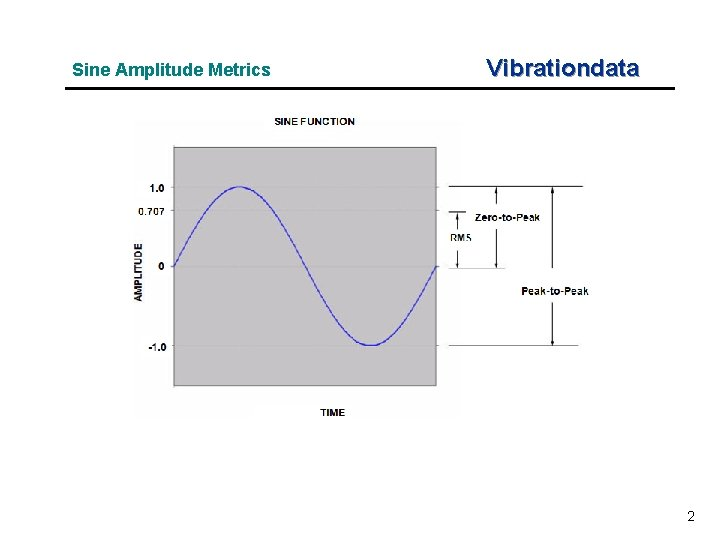Sine Amplitude Metrics Vibrationdata 2Question Vibrationdata Does sinusoidal vibration ever occur in rocket vehicles? 3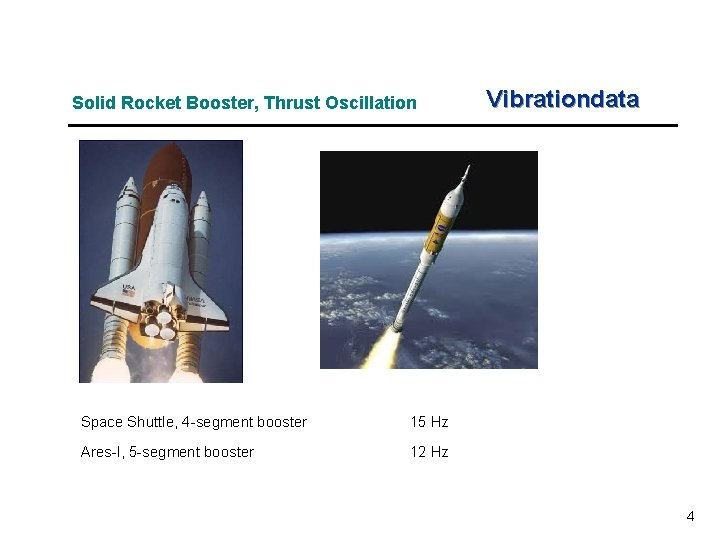Solid Rocket Booster, Thrust Oscillation Space Shuttle, 4 -segment booster 15 Hz Ares-I, 5 -segment booster 12 Hz Vibrationdata 4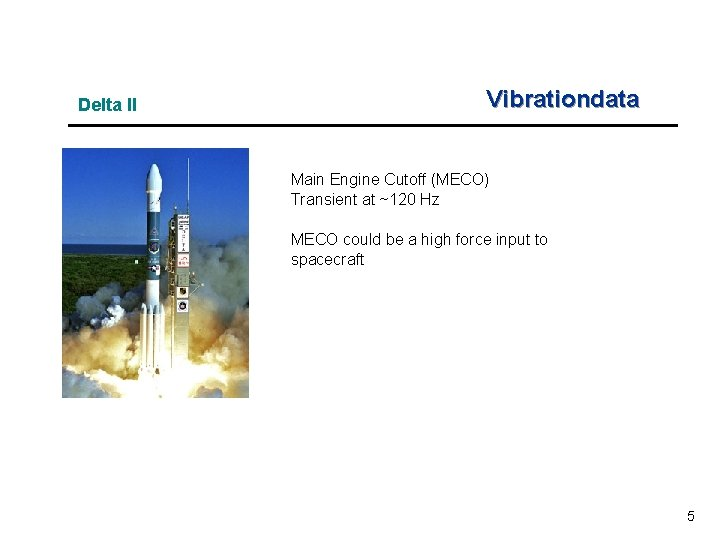Delta II Vibrationdata Main Engine Cutoff (MECO) Transient at ~120 Hz MECO could be a high force input to spacecraft 5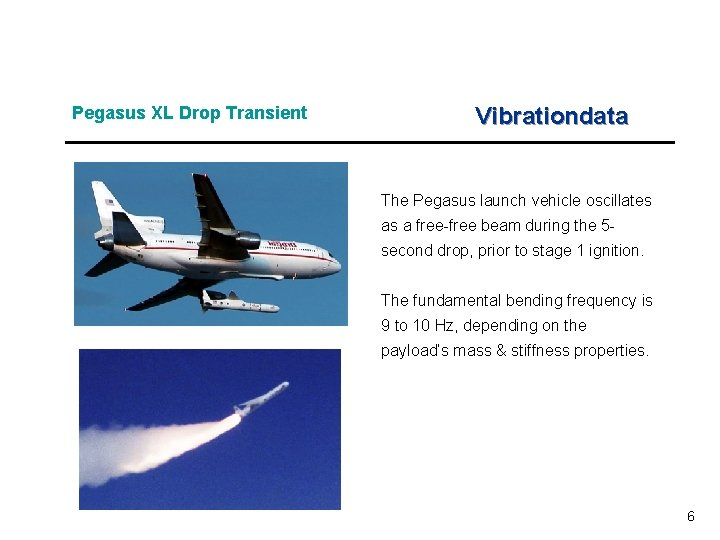Pegasus XL Drop Transient Vibrationdata The Pegasus launch vehicle oscillates as a free-free beam during the 5 second drop, prior to stage 1 ignition. The fundamental bending frequency is 9 to 10 Hz, depending on the payload’s mass & stiffness properties. 6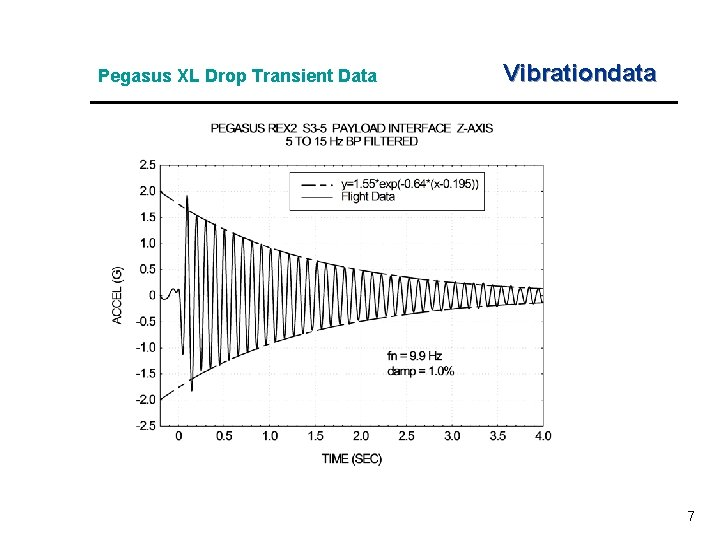Pegasus XL Drop Transient Data Vibrationdata 7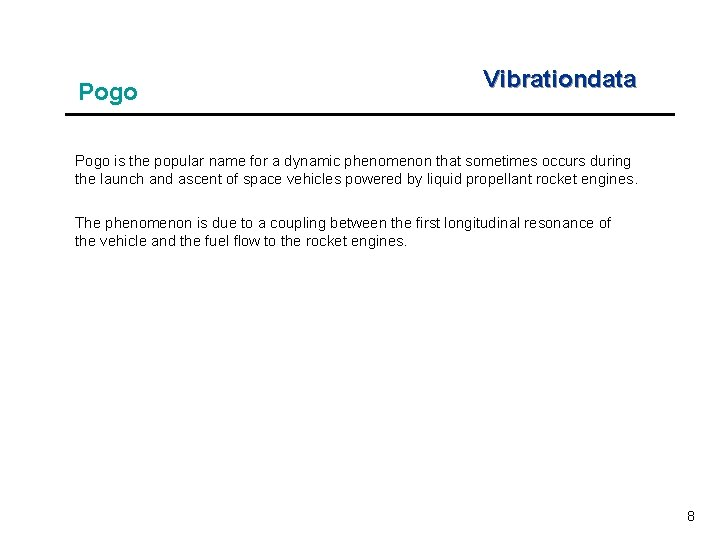Pogo Vibrationdata Pogo is the popular name for a dynamic phenomenon that sometimes occurs during the launch and ascent of space vehicles powered by liquid propellant rocket engines. The phenomenon is due to a coupling between the first longitudinal resonance of the vehicle and the fuel flow to the rocket engines. 8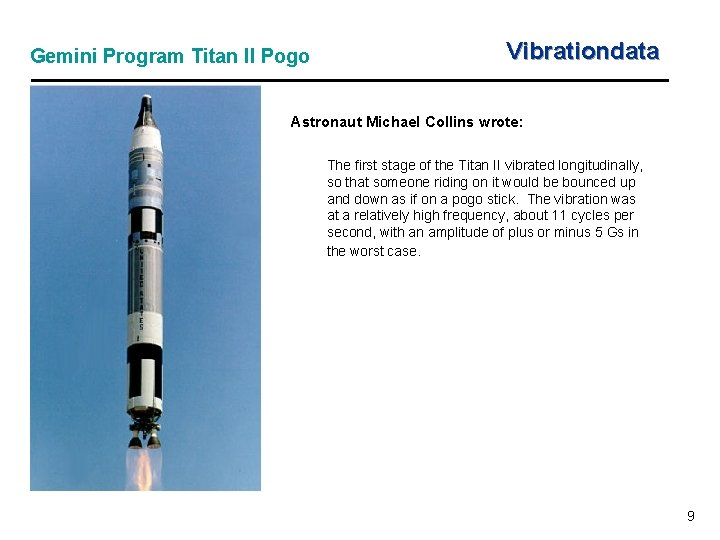Gemini Program Titan II Pogo Vibrationdata Astronaut Michael Collins wrote: The first stage of the Titan II vibrated longitudinally, so that someone riding on it would be bounced up and down as if on a pogo stick. The vibration was at a relatively high frequency, about 11 cycles per second, with an amplitude of plus or minus 5 Gs in the worst case. 9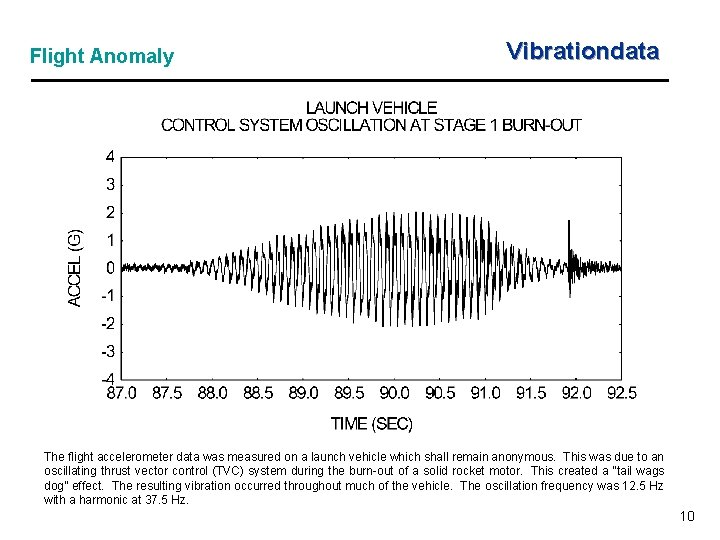Flight Anomaly Vibrationdata The flight accelerometer data was measured on a launch vehicle which shall remain anonymous. This was due to an oscillating thrust vector control (TVC) system during the burn-out of a solid rocket motor. This created a “tail wags dog” effect. The resulting vibration occurred throughout much of the vehicle. The oscillation frequency was 12. 5 Hz with a harmonic at 37. 5 Hz. 10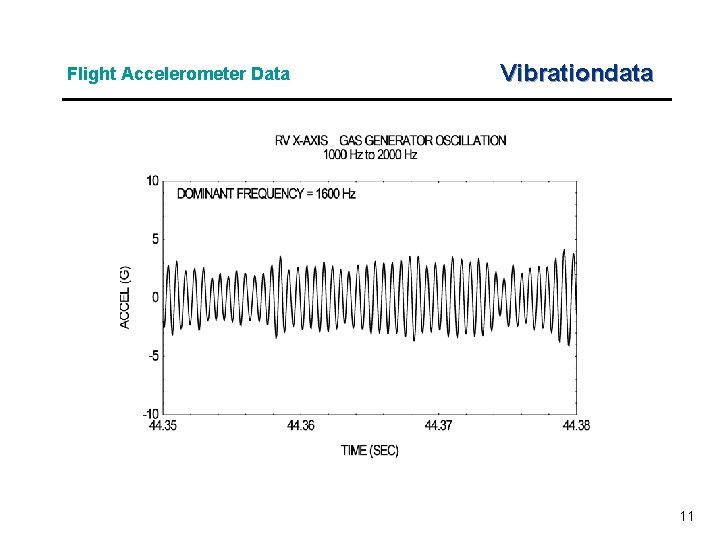Flight Accelerometer Data Vibrationdata 11Sine Function Example Vibrationdata 12Sine Function Bathtub Histogram Vibrationdata 13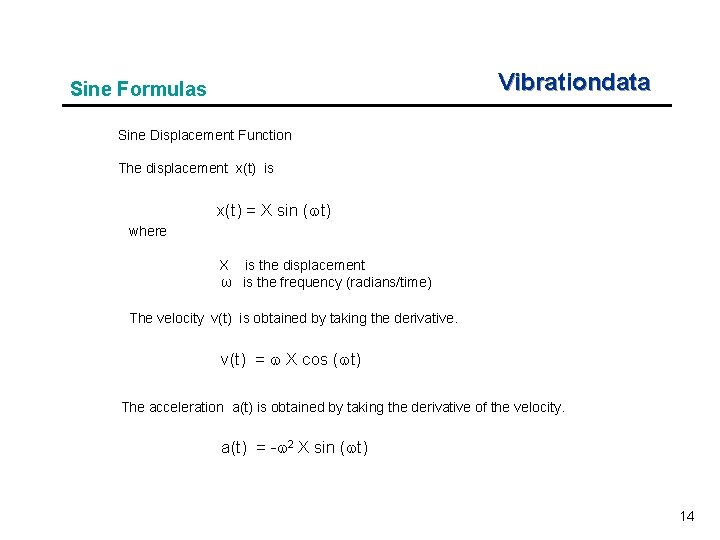Vibrationdata Sine Formulas Sine Displacement Function The displacement x(t) is x(t) = X sin ( t) where X is the displacement ω is the frequency (radians/time) The velocity v(t) is obtained by taking the derivative. v(t) = X cos ( t) The acceleration a(t) is obtained by taking the derivative of the velocity. a(t) = - 2 X sin ( t) 14Vibrationdata Peak Sine Values Peak Values Referenced to Peak Displacement Parameter Value displacement X velocity X acceleration 2 X Peak Values Referenced to Peak Acceleration Parameter acceleration Value A velocity A/ displacement A/ 2 15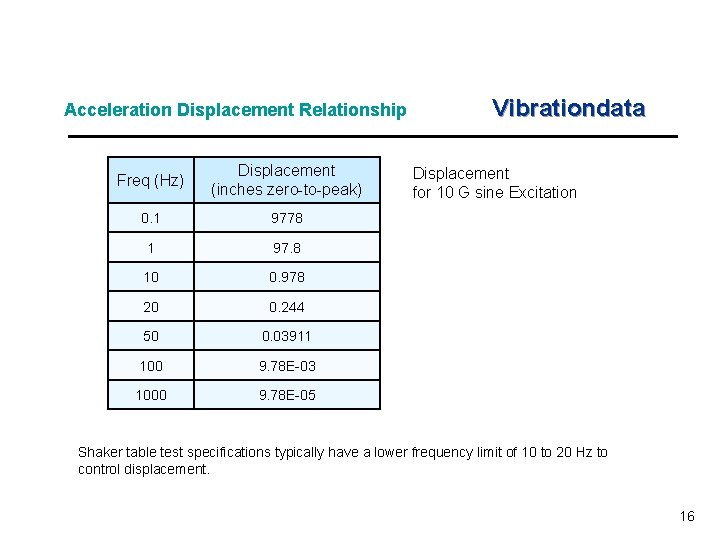Acceleration Displacement Relationship Freq (Hz) Displacement (inches zero-to-peak) 0. 1 9778 1 97. 8 10 0. 978 20 0. 244 50 0. 03911 100 9. 78 E-03 1000 9. 78 E-05 Vibrationdata Displacement for 10 G sine Excitation Shaker table test specifications typically have a lower frequency limit of 10 to 20 Hz to control displacement. 16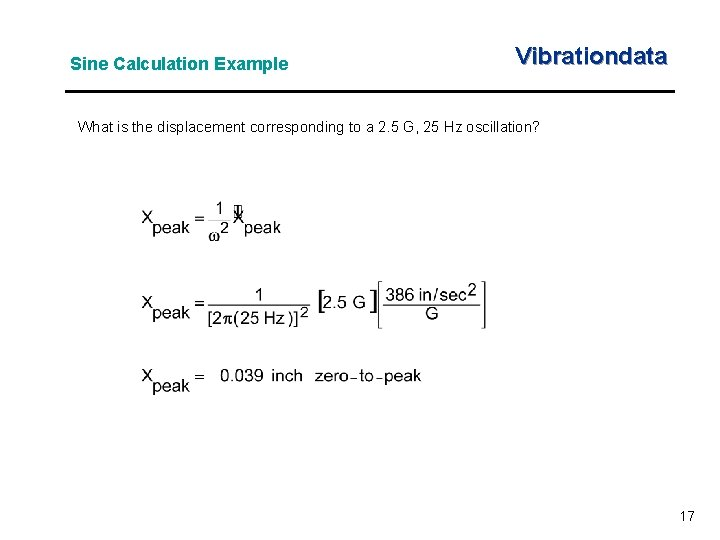Sine Calculation Example Vibrationdata What is the displacement corresponding to a 2. 5 G, 25 Hz oscillation? 17Sine Amplitude Vibrationdata Sine vibration has the following relationships. These equations do not apply to random vibration, however. 18SDOF System Subjected to Base Excitation Vibrationdata 19Free Body Diagram Vibrationdata Summation of forces in the vertical direction Let z = x - y. The variable z is thus the relative displacement. Substituting the relative displacement yields 20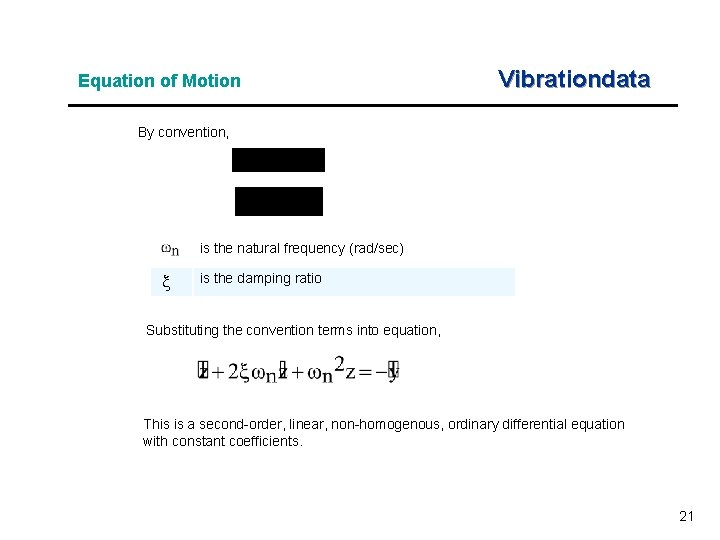Equation of Motion Vibrationdata By convention, is the natural frequency (rad/sec) is the damping ratio Substituting the convention terms into equation, This is a second-order, linear, non-homogenous, ordinary differential equation with constant coefficients. 21Equation of Motion (cont) Vibrationdata could be a sine base acceleration or an arbitrary function Solve for the relative displacement z using Laplace transforms. Then, the absolute acceleration is 22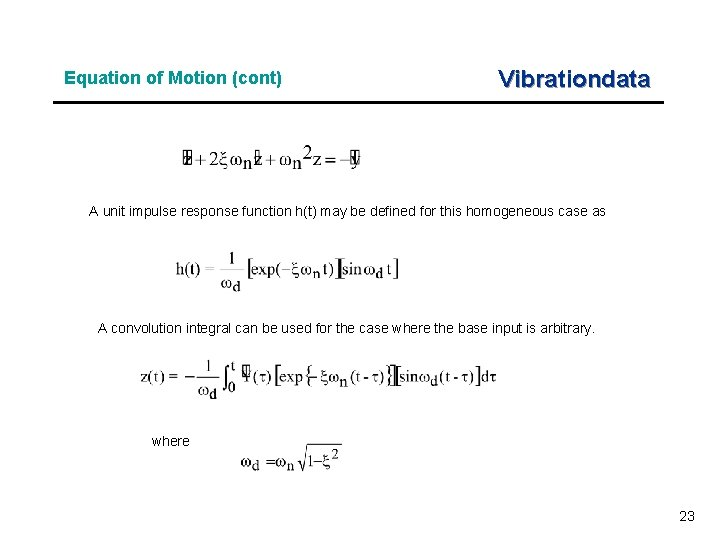Equation of Motion (cont) Vibrationdata A unit impulse response function h(t) may be defined for this homogeneous case as A convolution integral can be used for the case where the base input is arbitrary. where 23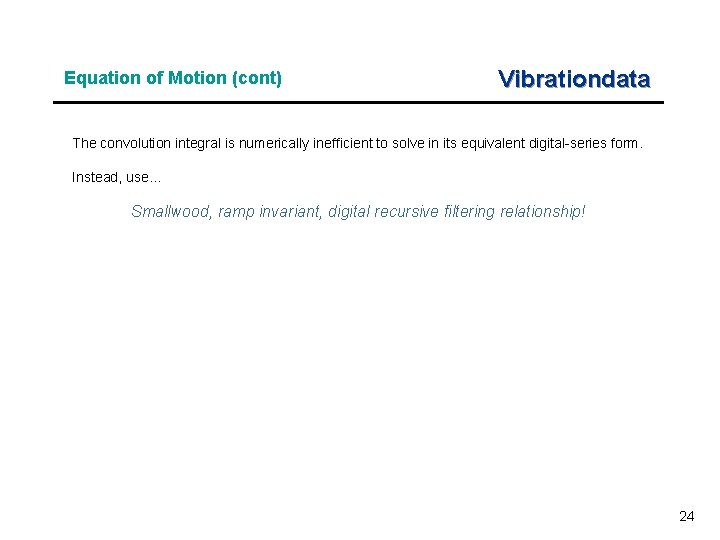Equation of Motion (cont) Vibrationdata The convolution integral is numerically inefficient to solve in its equivalent digital-series form. Instead, use… Smallwood, ramp invariant, digital recursive filtering relationship! 24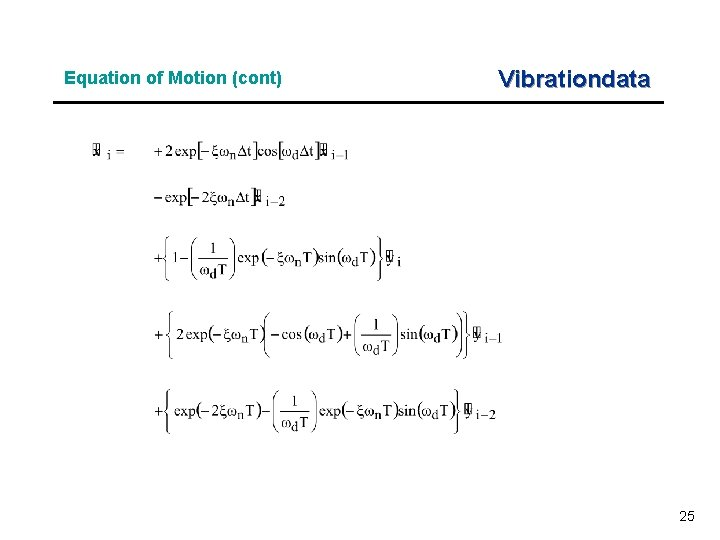Equation of Motion (cont) Vibrationdata 25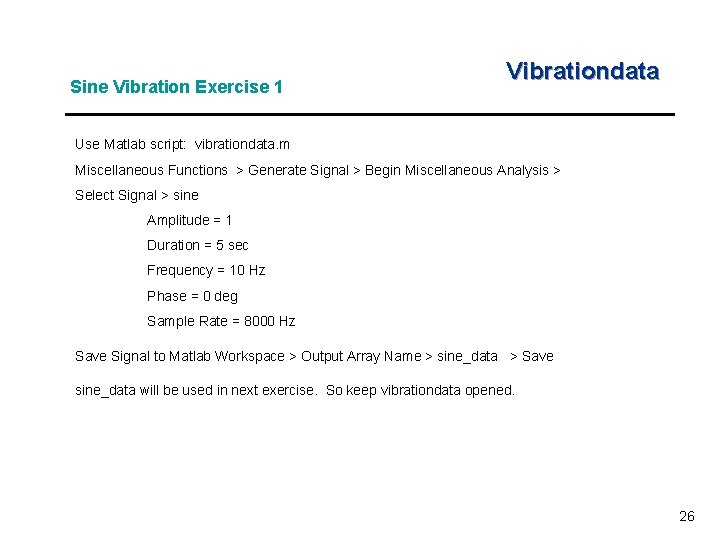Sine Vibration Exercise 1 Vibrationdata Use Matlab script: vibrationdata. m Miscellaneous Functions > Generate Signal > Begin Miscellaneous Analysis > Select Signal > sine Amplitude = 1 Duration = 5 sec Frequency = 10 Hz Phase = 0 deg Sample Rate = 8000 Hz Save Signal to Matlab Workspace > Output Array Name > sine_data > Save sine_data will be used in next exercise. So keep vibrationdata opened. 26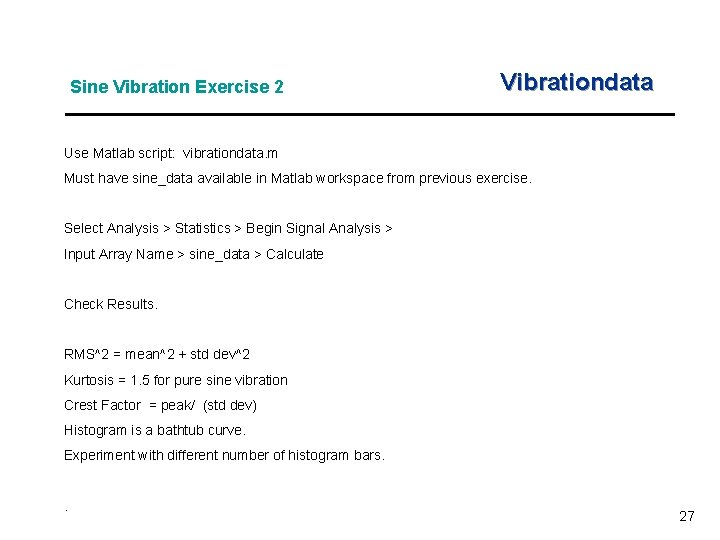Sine Vibration Exercise 2 Vibrationdata Use Matlab script: vibrationdata. m Must have sine_data available in Matlab workspace from previous exercise. Select Analysis > Statistics > Begin Signal Analysis > Input Array Name > sine_data > Calculate Check Results. RMS^2 = mean^2 + std dev^2 Kurtosis = 1. 5 for pure sine vibration Crest Factor = peak/ (std dev) Histogram is a bathtub curve. Experiment with different number of histogram bars. . 27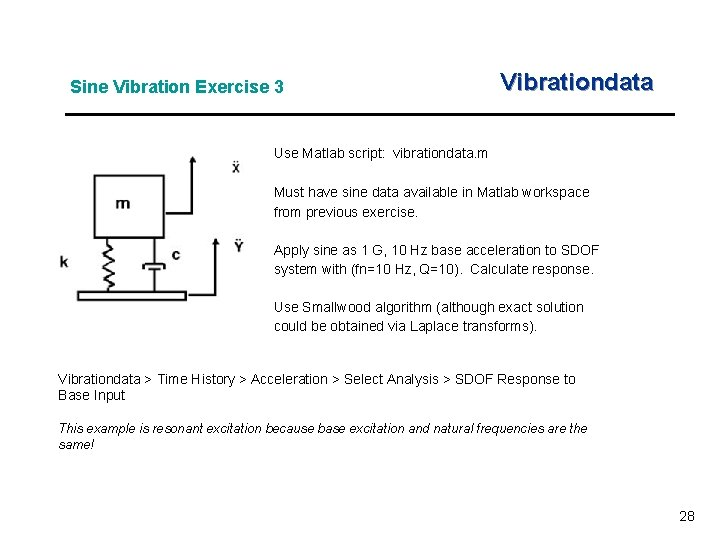Sine Vibration Exercise 3 Vibrationdata Use Matlab script: vibrationdata. m Must have sine data available in Matlab workspace from previous exercise. Apply sine as 1 G, 10 Hz base acceleration to SDOF system with (fn=10 Hz, Q=10). Calculate response. Use Smallwood algorithm (although exact solution could be obtained via Laplace transforms). Vibrationdata > Time History > Acceleration > Select Analysis > SDOF Response to Base Input This example is resonant excitation because base excitation and natural frequencies are the same! 28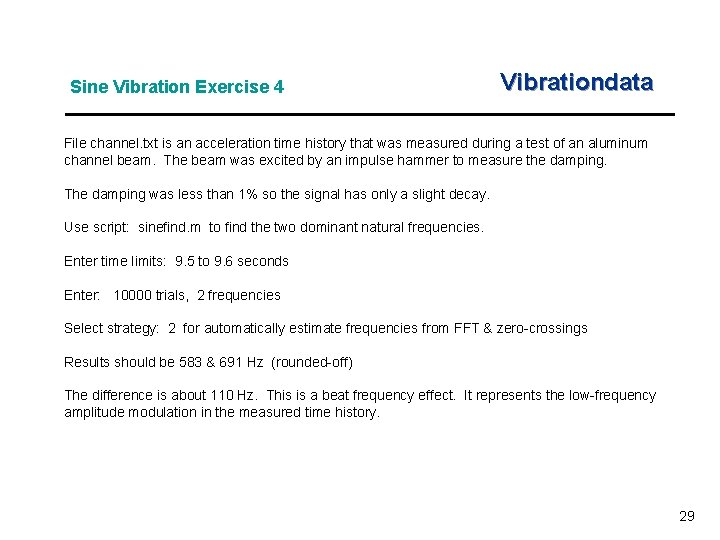Sine Vibration Exercise 4 Vibrationdata File channel. txt is an acceleration time history that was measured during a test of an aluminum channel beam. The beam was excited by an impulse hammer to measure the damping. The damping was less than 1% so the signal has only a slight decay. Use script: sinefind. m to find the two dominant natural frequencies. Enter time limits: 9. 5 to 9. 6 seconds Enter: 10000 trials, 2 frequencies Select strategy: 2 for automatically estimate frequencies from FFT & zero-crossings Results should be 583 & 691 Hz (rounded-off) The difference is about 110 Hz. This is a beat frequency effect. It represents the low-frequency amplitude modulation in the measured time history. 29Sine Vibration Exercise 5 Vibrationdata Astronaut Michael Collins wrote: The first stage of the Titan II vibrated longitudinally, so that someone riding on it would be bounced up and down as if on a pogo stick. The vibration was at a relatively high frequency, about 11 cycles per second, with an amplitude of plus or minus 5 Gs in the worst case. What was the corresponding displacement? Perform hand calculation. Then check via: Matlab script > vibrationdata > Miscellaneous Functions > Amplitude Conversion Utilities > Steady-state Sine Amplitude 30Sine Vibration Exercise 6 Vibrationdata A certain shaker table has a displacement limit of 2 inch peak-to-peak. What is the maximum acceleration at 10 Hz? Perform hand-calculation. Then check with script: vibrationdata > Miscellaneous Functions > Amplitude Conversion Utilities > Steady-state Sine Amplitude 31